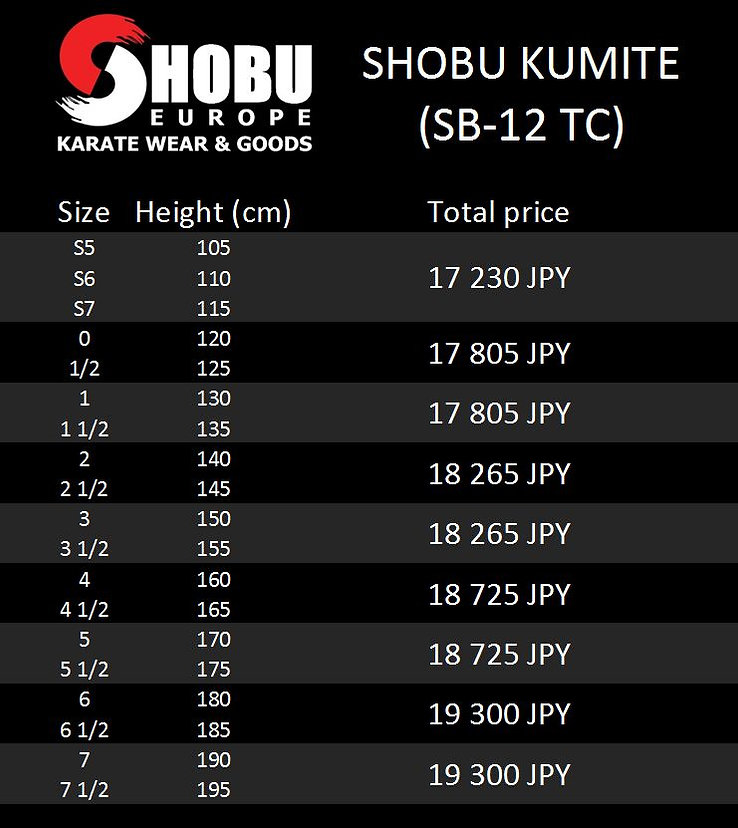We use XE.com to calculate the conversions - you can calculate the price in your currency by clicking here:We use XE.com to calculate the conversions - you can calculate the price in your currency by clicking here:We use XE.com to calculate the conversions - you can calculate the price in your currency by clicking here:We use XE.com to calculate the conversions - you can calculate the price in your currency by clicking here:We use XE.com to calculate the conversions - you can calculate the price in your currency by clicking here:We use XE.com to calculate the conversions - you can calculate the price in your currency by clicking here: